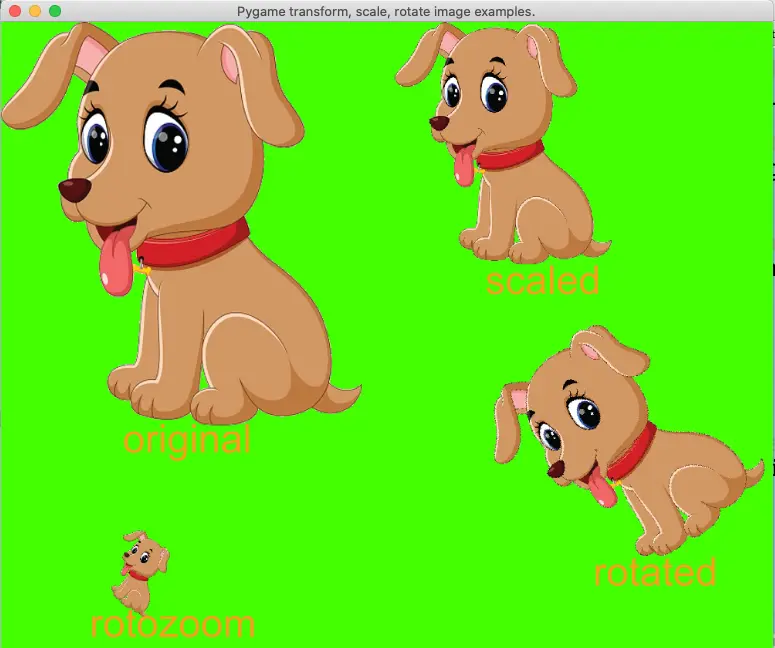# Pygame Transform, Rotate, And Scale Image Example

This article will tell you how to transform, rotate, scale, and rotate-zoom an image in Pygame with examples.

### 1. How To Scale A Surface Object ( an image) In Pygame.

1. The function pygame.transform.scale(surface, size, dest_surface=None) can scale a surface object ( for example an image ) to a provided size and return the new size surface object.
2. Below is the pygame’s scale() function example source code.
`scaled_image = pygame.transform.scale(src_img_surface,(300,300))`

### 2. How To Rotate A Surface Object ( an image ) In Pygame.

1. The function pygame.transform.rotate(surface, angle) can rotate the surface object with the provided angle, it will return a new surface object that has a rotate angle.
2. Below is the pygame’s rotate() function example source code.
`rotated_image = pygame.transform.rotate(src_img_surface, 36)`

### 3. How To Rotate & Scale A Surface Object ( an image ) At The Same Time In Pygame.

1. The function pygame.transform.rotozoom(surface, angle, scale) can implement both rotate and scale operation to the provided surface object ( an image object ), it will return the rotated & zoomed surface object.
2. Below is the pygame’s rotozoom() function example source code.
`zommed_img = pygame.transform.rotozoom(src_img_surface, -72, 0.3)`

### 4. How To Rotate, Scale Image In Pygame Example.

1. This example shows how to scale, rotate, zoom & rotate the surface object ( an image object ) in pygame application.
2. Below is the example source code, you can read the comments for a detailed explanation. The python source file name is PygameTransfromScaleRotateImage.py.
```'''
Created on Feb 6, 2022

@author: songzhao
'''

import pygame

# define a global variable to store the pygame app main window surface.
main_window_screen = None

# this function will initialize the pygame main window.
def initialize_pygame():

# initialize pygame app.
pygame.init()

# set pygame window title.
window_title = 'Pygame transform, scale, rotate image examples.'
pygame.display.set_caption(window_title)

# declare that the main_window_screen is a global variable.
global main_window_screen
# create the pygame main window surface object with provided size, the window is resizable.
main_window_screen = pygame.display.set_mode((1000,800), pygame.RESIZABLE)
# fill the pygame main window background color to green.
main_window_screen.fill(pygame.Color('green'))

# this function will load an image to the pygame app main window.

image_file_path = '../../../../img/dog.png'

# load an image object from the image file path.

# call the convert_alpha() function to make the image background alpha transparency.
image_surface_object = image_surface_object.convert_alpha()

# draw the image surface object to the main window screen.
update_screen(main_window_screen, image_surface_object, 0, 0)

return image_surface_object

# this function will scale the image surface object.
def scale_image(src_img_surface):

# scale the source image surface object to a new size.
scaled_image = pygame.transform.scale(src_img_surface,(300,300))

# draw the scaled image surface object to the pygame app main window screen.
update_screen(main_window_screen, scaled_image, src_img_surface.get_width()+10, 0)

return scaled_image

# this function will rotate the image surface object.
def rotate_image(src_img_surface):

# rotate the source image surface object with a provided angle (36 in this example).
rotated_image = pygame.transform.rotate(src_img_surface, 36)

# calculate the rotated image x, y coordinates.
x = main_window_screen.get_width() - src_img_surface.get_width() - 100
y = src_img_surface.get_height() + 10

# draw the rotated image to the pygame app main window screen.
update_screen(main_window_screen, rotated_image, x, y)

return rotated_image

# this function can rotate and scale (zoom) the image at the same time.
def zoom_image(src_img_surface):

# rotate and zoom the image with the provided parameters at the same time.
zommed_img = pygame.transform.rotozoom(src_img_surface, -72, 0.3)

# calculate the new image surface object x, y coordinates.
x = 100
y = main_window_screen.get_height() - 200

# draw the zoomed_img to the pygame app main window.
update_screen(main_window_screen, zommed_img, x, y)

return zommed_img

# this function listen and handle the pygame app events.
def main_loop():

while True:

for event in pygame.event.get():

if event.type == pygame.QUIT:

exit()

# this function will draw the target_surface_object to the source surface_object at the specified location.
def update_screen(surface_object, target_surface_object, top_left_corner_x, top_left_corner_y):

surface_object.blit(target_surface_object,(top_left_corner_x, top_left_corner_y))

pygame.display.update()

if __name__ == '__main__':

initialize_pygame()